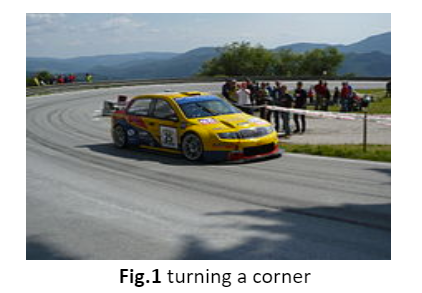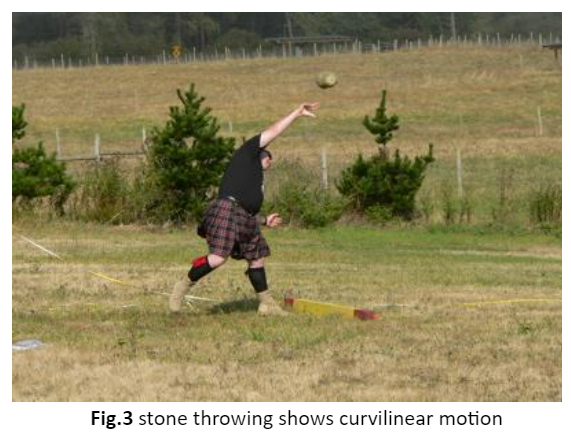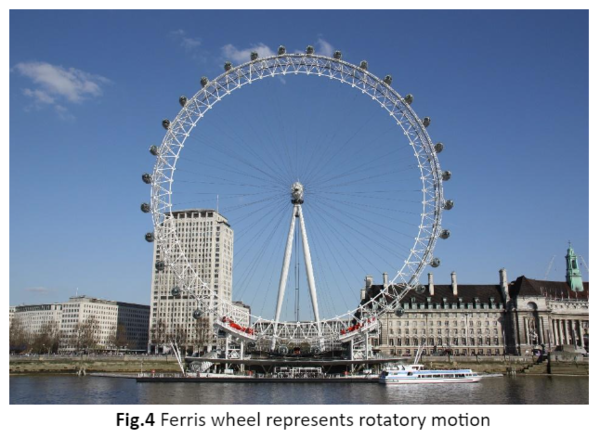• ### Browse All Lessons

##### Assign Lesson

Help Teaching subscribers can assign lessons to their students to review online!

 Tweet# Types of Motion

This lesson aligns with Next Generation Science Standards (NGSS) PS2.A

Introduction
A body is said to be in motion if its distance changes from another object. In other words, motion is a change of position of an object corresponding to time. Everything is in a state of continuous motion. Examples include dust falling from the carpet, a fan, moving vehicles on the road, etc. Other activities such as walking, running, and eating possess motion as well. Motion is mainly categorized into three types that are linear motion, rotatory motion, and oscillatory motion. In this article, we will learn about types of motion, linear motion, rotatory motion, and oscillatory motion.

Types of Motion
Every moving object possesses some kind of motion. For instance, a car starts moving from the rest position to a straight line, and after some time interval, it takes a turn and continues its motion on a curve path. Then it climbs up a hill and reaches its destination following the zig-zag path. During a complete trip, a car experiences different motions on different tracks.Similarly, different objects move differently. Based on the nature of the movement, motion is mainly categorized into three types that are;
• Linear motion
• Rotatory motion
• Oscillatory motion

Linear Motion
Linear motion is the most fundamental and natural to an object, e.g., moving in a straight line. An object possesses linear motion that moves from one position to another, either in a straight line or curve path. Linear motion is further divided into two types depending on the path of the motion.

Rectilinear Motion
The rectilinear motion is a linear motion in which the velocity remains constant. The trajectory is a straight line. The motion of a vehicle on a road and a bullet fired from a gun are examples of rectilinear motion.Curvilinear motion
It is defined as the motion of an object traveling along a curve path. The velocity of the object is always tangent to the path during curvilinear motion. For example, when a stone is thrown into the air at some angle, it represents the curvilinear motion.Rotatory Motion
The rotatory motion refers to the motion in which the object rotates around a fixed axis. The rotation of the earth and other planets about their respective axis is an example of rotatory motion. A blender is a common example of rotatory motion.

A Ferris wheel is an amusement ride and a major attraction of any funfair. It consists of a huge metallic wheel which consists of several cabins to carry the people in it. When the power supply is on, the wheel rotates about its central point. Thus, the Ferris wheel represents rotatory motion in real life.Oscillatory Motion
The oscillatory motion refers to the motion in which the object repeats its motion about its mean position. In such a type of motion, an object moves in such a way that it comes to and goes away from the mean position periodically. It is also known as vibratory motion. The movement of a simple pendulum in a clock demonstrates oscillatory motion as it moves to and fro about its mean position.

Swing also represents oscillatory motion. When the force is applied to the swing, it moves to the extreme position in a forward direction and then returns to the mean position. It does not stop here and again moves to the extreme position in the backward direction. It moves to and fro about its mean position.Periodic Motion
The motion which repeats itself after equal intervals of time is known as periodic motion. It also classifies as oscillatory motion. For instance, a tuning fork and a simple pendulum. The time taken by the motion of an object to repeat itself is known as the time period. The S.I. unit of time period is second. Frequency refers to the number of times the motion is repeated in one second. Frequency is expressed in Hz or Hertz.

Summary
• We often notice that different objects move differently. According to the nature of the movement, motion is further divided into three types.
• The object moves from one position to another, either in a straight line or curved path, exhibiting linear motion.
• Rectilinear motion means the path of the motion is a straight line, while curvilinear motion means the object moves on a curved path.
• Rotatory motion refers to the motion of an object when it rotates or spins about its axis. Some examples are fan blades, tuning forks, spinning tops, etc.
• When an object moves to and fro about its mean position, then the object is said to be in exhibitory oscillatory motion.

Related Worksheets: